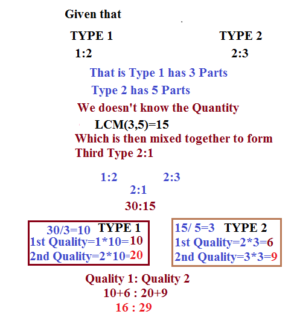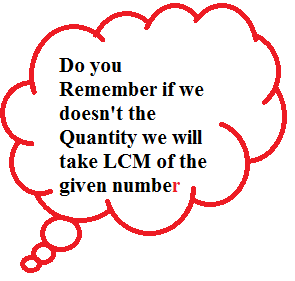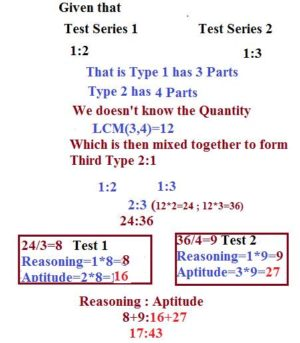# How to solve Ratio and Proportion – Tougher Problems

Hi Bankersdaily Aspirants,

Today we are going to look,how to solve Ratio & Proportion in new HOW TO SERIES.Before looking into the topic let us look on Basics of Ratio & Proportion

PROBLEMS DISCUSSED BASED ON

1)MIXING 2 ALLOYS TO FORM A 3rd ALLOY

2)PRACTICE PROBLEM WITH EXPLANATION

3)HOW TO OBTAIN PERCENTAGE OF THE MIXTURE

4)PRACTICE PROBLEM WITH EXPLANATION

## #.1.TYPE 1:

### MIXING 2 ALLOYS TO FORM A 3rd ALLOY:

The Question will contain the 2 Ratio’s of Alloys & they will specify the 3rd Ratio in which they are going to mix the 2 Alloys & they ask us to find the individual capacity of each Alloy.Let us Solve to get better Understanding.

1)Vendor sells two Types of Rice Variety by mixing two qualities of rice in the ratio 1:2 & 2:3 and they are mixed to form the third Type in the Ratio 2:1.Find Out the Ratio of the individual quality of Rice after mixing?

EXPLANATION### 2)PRACTICE PROBLEM WITH EXPLANATION:

1)A Milk Vendor has  Milk and Water in the Ratio 3:5 in 1 Can &  Milk and Water in the Ratio 3:7 in 2nd Can. and he Pour these 2 cans into one can and there by his Ratio becomes 4:5.Find the Ratio of Milk & Water in the New Can.

Ratio in Can 1         Ratio in Can 2

3:5                              3:7

Therefore there is 8 Parts in Can 1 &

10 Parts in Can 2.

Ratio in Third Can=4:5

Since we doesn’t know the Quantity therefore Quantity is LCM of given Parts

LCM(8,10)=40 litres

Third can is in the Ratio 4:5

4*40=160 Litres

5*40=200 Litres

## 160 : 200

Can 1 has Milk & Water in the Ratio 3x:5x=160/8,x=20

3x=60 5x=100

Can 2 has Milk and water in the Ratio 3x+7x=10x=200;x=20

3x=60 &  7x=140

There the Milk & Water in the Ratio

60+60 : 100+140

120:240

1:2

## #.2 TYPE 2:

### HOW TO OBTAIN PERCENTAGE OF THE MIXTURE:

In the above type we had found the individual capacity of the Mixture which is asked in the exam but you all know nowadays question paper becomes so tougher they may ask us to find the percentage of the mixture.So  let us see how to calculate the Percentage of the mixture which may ask in the exam.

1)Test Series is provided to the students who are registered in simbatech and the series contains 2 Sections Reasoning & Aptitude. In 1st Test Series the Ratio of Reasoning & Aptitude Question asked is 1:2 and In 2nd Test Series the Ratio of Reasoning &  Aptitude Questions Asked is 1:3 & in the Final Series they combine these two test Series and the questions are taken in the Ratio 2:3.Then,Find the Percentage of Aptitude Section in the test Series?

Explanation### TO CALCULATE PERCENTAGE:

Percentage of Questions in Aptitude Section=(43/60)*100

Aptitude Question=43

Total Question=60(43+17)